Page 2
Go to:  P1     P3   P4 (data)OFFSET NULL
Many OP-AMPs have two pins labeled OFFSET NULL. When both inputs are connected to the same voltage, the output should be zero. If the project requires a zero output under these conditions, the OFFSET NULL should be adjusted by adding a 10k pot between the Offset Null pins with the centre of the pot connected to 0v.
By adjusting the pot, the output will produce 0v.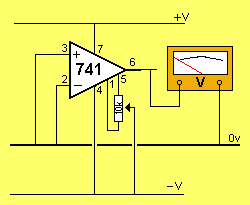NON-INVERTING AMPLIFIER
The circuit shows an OP-AMP connected as a NON-INVERTING AMPLIFIER:INVERTING AMPLIFIER
The circuit shows an OP-AMP connected as an INVERTING AMPLIFIER:THE OP-AMP AS A VOLTAGE FOLLOWER
The circuit shows an OP-AMP connected as a VOLTAGE FOLLOWER:THE OP-AMP AS A COMPARATOR
The OP-AMP can compare two signals (voltages). This is called a COMPARATOR or DIFFERENTIAL AMPLIFIER (amplifies the difference between two signals).
There are two arrangements - connection to a single rail or dual rails. The animations below show the output for each configuration: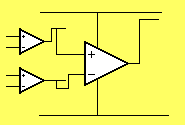THE OP-AMP AS A SCHMITT TRIGGER
The OP-AMP can be wired as a Schmitt trigger. The diagram below shows this arrangement: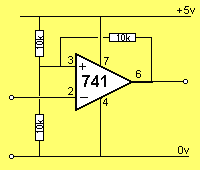When the input of the Schmitt Trigger is LOW, the output is HIGH.
As the input rises, nothing happens to the output until the input is 3v3. This is the voltage on the "+" input due to the effect of the three 10k resistors. These 3 resistors form a voltage divider with two 10k resistors connected to the 5v supply and one 10k resistor connected to 0v.
When the "–" input is 3v3, the output of the OP-AMP goes LOW and it remains LOW until the input falls to less than 1v6. The 1v6 voltage on the "+" input is produced by the three 10k resistors. When the output is LOW, one 10k resistor is connected to the 5v supply and two resistors are connected to the 0v rail. This produces 1v6 on the "+" input.
The purpose of a Schmitt Trigger is to detect and respond to a signal that rises and falls a large amount - in other words it has "large excursions."
There are also signals that rise and fall very slowly - such as a photo transistor detecting daylight.
During the detection process, the output will rise and fall slightly during the morning light and the change from one level to the other will cause the project to turn on and off.
This is unwanted. The Schmitt trigger will produce an output when a definite condition is met and will not change until the daylight is reduced considerably.

 PRACTICAL CIRCUITS
Here are some practical circuits using OP-AMPS: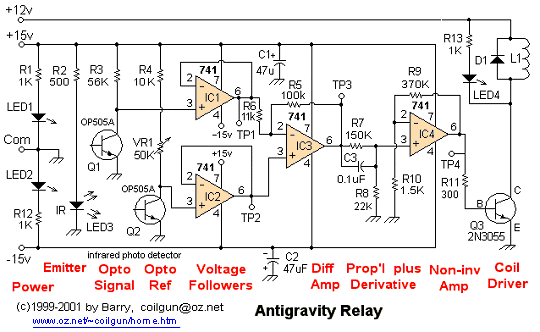TIMER
When the push-button is pressed and released, the LED illuminates after a period of time. The heart of the circuit is an OP-AMP configured as a comparator. The operation is as follows. When the voltage at "+" input is less than the voltage at "–" input, the output at the output is LOW. When voltage at "+" input is more than the voltage at "–" input, output is HIGH.  It is usual to hold the voltage at "–" input at a particular voltage, known as the reference voltage, and vary the voltage at "+" input to obtain a particular function. The two 10k resistors connected in series form a voltage divider, the voltage at the mid-point being 4.5v
The 500k pot sets the time for the 2200u to charge above 4v5. The 1k stop-resistor prevents a short-circuit if the pot is set to minimum resistance and the button is pressed.
Pressing the switch resets the circuit.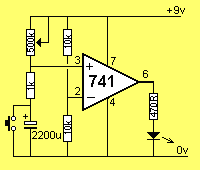SIMPLE INTERCOM
A simple intercom can be built around an OP-AMP: